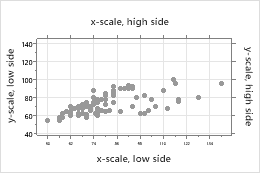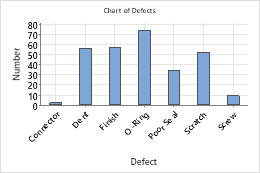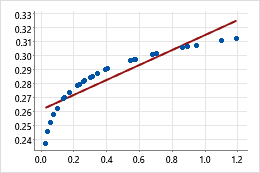# Continuous scale

This topic is about continuous graph scales, such as the axes on a scatterplot. For information on other scale types, click one of the following links.

## Show or hide scale elements

Graph scale elements are axis lines, axis labels, tick marks, and tick labels. You can display these elements on the high side or the low side of the graph's data region.

For example, on the following scatterplot, major and minor tick marks and tick labels are displayed on the low sides of the graph. Major tick marks are displayed on the high sides of the graph. Axis lines are on all sides.To specify which elements to include when you create a graph, use the following steps:
1. Click Scale.
2. On the Axes and Ticks tab, select the elements to show.
To change or add the elements on an existing graph, use the following steps:
1. Double-click the graph.
2. Double-click the scale to open the Edit Scale dialog box.
3. On the Show tab, select the elements to show.

## Transpose the y-scale and x-scale

For probability plots, bar charts, boxplots, interval plots, individual values plots, and histograms, you can transpose the horizontal and vertical scales.Example of transposing the scalesIn a default bar chart, the categorical scale is horizontal and the continuous scale is vertical. To emphasize the differences in the values, you can transpose the two scales.
To transpose the y- and x-scales when you create a graph, use the following steps:
1. Click Scale.
2. On the Axes and Ticks tab, select the option to transpose the scales.
To transpose scales on an existing graph, use the following steps:
1. Double-click the graph.
2. Double-click the scale to open the Edit Scale dialog box.
3. On the Scale tab, select the option to transpose the scales.

## Do not adjust the scale with the threshold parameter

(Probability plots only) By default, if you select a distribution that has a threshold parameter, Minitab adjusts the x-scale values by subtracting the threshold parameter. This adjustment straightens the fitted distribution line. To plot the unadjusted x-values when you create a graph, use the following steps:
1. Click Scale.
2. On the Axes and Ticks, deselect Adjust scale for threshold if distribution has this parameter.
To deselect this option for an existing graph, use the following steps:
1. Double-click the graph.
2. Double-click the scale to open the Edit Scale dialog box.
3. On the Scale tab, deselect Adjust scale for threshold if distribution has this parameter.

## Change the position of the major tick marks

When you create a graph, Minitab sets the tick mark positions to fit the scale. To change the major tick mark positions on an existing graph, use the following steps:
1. Double-click the graph.
2. Double-click the scale to open the Edit Scale dialog box.
3. On the Scale tab, under Major Tick Positions, specify one of the following:
• Automatic: Display the tick marks in their default positions.
• Number of ticks: Enter the number of tick marks. Minitab spaces the tick marks evenly along the axis line. (Not available for all graphs.)
• Position of ticks: Enter the scale values at which you want major tick marks to appear. You can enter a series of numbers or specify a range of values. Use a colon between two numbers to indicate the range. Use a slash after the range to indicate an increment amount. For example, 5:30/5 places tick marks at 5, 10, 15, 20, 25, and 30.
For example, the following scatterplots illustrate the same data. One plot shows the default tick mark positions and the other plot uses custom tick mark positions.
###### Tip

To change the bin widths for the x-scale on graphs with bins, click the Binning tab. For more information, go to Binning.

## Change the scale range

Minitab sets the scale range so that the observations extend the length of the axis. To compress or expand the display of observations on the axis, use the following steps:
1. Double-click the graph.
2. Double-click the scale to open the Edit Scale dialog box.
3. On the Scale tab, under Scale Range, deselect Auto for Minimum or Maximum, then enter the new minimum or maximum.
For example, the following boxplots are of the same data, but the y-scale ranges are different.

## Change the number of minor tick marks

To change the number of minor tick marks that appear between two major tick marks, use the following steps:
1. Double-click the graph.
2. Double-click the scale to open the Edit Scale dialog box.
3. On the Scale tab, deselect Auto, then enter a number.
###### Note

For minor tick marks to appear on the graph, you must select them on the Show tab.

## Change the axis line attributes and tick orientation

To change the color, size, and line type of the axis lines and tick marks, and the orientation of tick marks and the length of major tick marks, use the following steps:
1. Double-click the graph.
2. Double-click the scale to open the Edit Scale dialog box.
3. On the Attributes tab, specify the options.
The following images illustrate the tick orientation.

## Change the tick mark labels

When you create a graph, Minitab uses data values as tick mark labels. To specify custom tick mark labels on an existing graph, use the following steps:
1. Double-click the graph.
2. Double-click the scale to open the Edit Scale dialog box.
3. On the Labels tab, select Specified, then enter custom labels, separated by spaces.
For example, on the first graph, the default labels are rotated because they are too long to fit. The shorter custom labels on the second graph fit better.

Edit the font for the tick mark labels on the Font tab. Change the angle of tick mark labels on the Alignment tab.

## Change the axis labels

To edit an axis label, use the following steps:
1. Double-click the graph.
2. Double-click the axis label to open the Edit Axis Label dialog box.
3. Select the tab to make the edits that you want.
• Font: Edit the text, and the font.
• Alignment: Specify the text angle and the position relative to the default location.
• Show: Specify the location on the graph where you want the label to display.
To edit the axis label text on a matrix plot, use the following steps:
1. Double-click the graph.
2. Double-click the label to open the Edit Axis Label dialog box.
3. On the Text tab, deselect Auto, and enter the custom text.

## Transform a continuous scale with a logarithm or power

On a scatterplot, time series plot, and area graph, you can transform a continuous scale with a logarithm or power to get a different view of your data.Example of transforming a scaleIn the original scatterplot, the simple fitted regression line does not accurately model the curvature in the data. When the horizontal scale is transformed to logarithmic values, the simple fitted regression line accurately models the data.
To transform the scale on a graph, use the following steps:
1. Double-click the graph.
2. Double-click the axis of the continuous scale to open the Edit Scale dialog box.
3. On the Transform tab, choose Logarithm or Power, and specify the parameters.
By using this site you agree to the use of cookies for analytics and personalized content.  Read our policy